# Resources tagged with: Calculating with fractions

Filter by: Content type:
Age range:
Challenge level:

### There are 44 results

Broad Topics > Fractions, Decimals, Percentages, Ratio and Proportion > Calculating with fractions### Once Upon a Time

##### Age 7 to 11 Challenge Level:

Can you work out the height of Baby Bear's chair and whose bed is whose if all the things the three bears have are in the same proportions?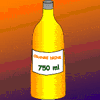### Orange Drink

##### Age 7 to 11 Challenge Level:

A 750 ml bottle of concentrated orange squash is enough to make fifteen 250 ml glasses of diluted orange drink. How much water is needed to make 10 litres of this drink?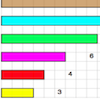### Fraction Lengths

##### Age 7 to 11 Challenge Level:

Can you find combinations of strips of paper which equal the length of the black strip? If the length of the black is 1, how could you write the sum of the strips?### A Chance to Win?

##### Age 11 to 14 Challenge Level:

Imagine you were given the chance to win some money... and imagine you had nothing to lose...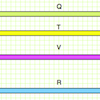### More Fraction Bars

##### Age 7 to 11 Challenge Level:

What fraction of the black bar are the other bars? Have a go at this challenging task!##### Age 7 to 11 Challenge Level:

Try adding fractions using A4 paper.### A4 Fraction Subtraction

##### Age 7 to 11 Challenge Level:

This task offers opportunities to subtract fractions using A4 paper.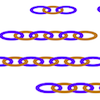##### Age 7 to 11 Challenge Level:

Can you find ways to make twenty-link chains from these smaller chains? This gives opportunities for different approaches.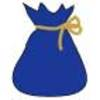### Andy's Marbles

##### Age 7 to 11 Challenge Level:

Andy had a big bag of marbles but unfortunately the bottom of it split and all the marbles spilled out. Use the information to find out how many there were in the bag originally.### Fun Time

##### Age 7 to 11 Challenge Level:

On Saturday, Asha and Kishan's grandad took them to a Theme Park. Use the information to work out how long were they in the theme park.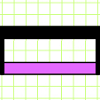### Extending Fraction Bars

##### Age 7 to 11 Challenge Level:

Can you compare these bars with each other and express their lengths as fractions of the black bar?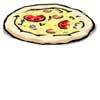### Pizza Portions

##### Age 7 to 11 Challenge Level:

My friends and I love pizza. Can you help us share these pizzas equally?##### Age 11 to 14 Challenge Level:

Aisha's division and subtraction calculations both gave the same answer! Can you find some more examples?### Tweedle Dum and Tweedle Dee

##### Age 11 to 14 Challenge Level:

Two brothers were left some money, amounting to an exact number of pounds, to divide between them. DEE undertook the division. "But your heap is larger than mine!" cried DUM...### Tray Bake

##### Age 11 to 14 Challenge Level:

My recipe is for 12 cakes - how do I change it if I want to make a different number of cakes?### The Greedy Algorithm

##### Age 11 to 14 Challenge Level:

The Egyptians expressed all fractions as the sum of different unit fractions. The Greedy Algorithm might provide us with an efficient way of doing this.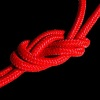### Twisting and Turning

##### Age 11 to 14 Challenge Level:

Take a look at the video and try to find a sequence of moves that will untangle the ropes.### Unit Fractions

##### Age 11 to 14 Challenge Level:

Consider the equation 1/a + 1/b + 1/c = 1 where a, b and c are natural numbers and 0 < a < b < c. Prove that there is only one set of values which satisfy this equation.### Fair Feast

##### Age 5 to 11 Challenge Level:

Here is a picnic that Petros and Michael are going to share equally. Can you tell us what each of them will have?### 3388

##### Age 11 to 14 Challenge Level:

Using some or all of the operations of addition, subtraction, multiplication and division and using the digits 3, 3, 8 and 8 each once and only once make an expression equal to 24.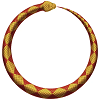### Snake Coils

##### Age 7 to 11 Challenge Level:

This challenge asks you to imagine a snake coiling on itself.### Jumping

##### Age 7 to 11 Challenge Level:

After training hard, these two children have improved their results. Can you work out the length or height of their first jumps?##### Age 11 to 14 Challenge Level:

Can you work out which drink has the stronger flavour?### Countdown Fractions

##### Age 11 to 16 Challenge Level:

Here is a chance to play a fractions version of the classic Countdown Game.### Peaches Today, Peaches Tomorrow...

##### Age 11 to 14 Challenge Level:

A monkey with peaches, keeps a fraction of them each day, gives the rest away, and then eats one. How long can his peaches last?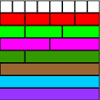##### Age 7 to 11 Challenge Level:

Use the fraction wall to compare the size of these fractions - you'll be amazed how it helps!### Pies

##### Age 7 to 11 Challenge Level:

Grandma found her pie balanced on the scale with two weights and a quarter of a pie. So how heavy was each pie?### Mathematical Swimmer

##### Age 11 to 14 Challenge Level:

Can you work out how many lengths I swim each day?### More Twisting and Turning

##### Age 11 to 16 Challenge Level:

It would be nice to have a strategy for disentangling any tangled ropes...##### Age 11 to 16 Challenge Level:

The items in the shopping basket add and multiply to give the same amount. What could their prices be?### In the Money

##### Age 7 to 11 Challenge Level:

One quarter of these coins are heads but when I turn over two coins, one third are heads. How many coins are there?### Fractions in a Box

##### Age 7 to 11 Challenge Level:

The discs for this game are kept in a flat square box with a square hole for each. Use the information to find out how many discs of each colour there are in the box.### Egyptian Fractions

##### Age 11 to 14 Challenge Level:

The Egyptians expressed all fractions as the sum of different unit fractions. Here is a chance to explore how they could have written different fractions.### Hello Again

##### Age 11 to 14 Challenge Level:

Anne completes a circuit around a circular track in 40 seconds. Brenda runs in the opposite direction and meets Anne every 15 seconds. How long does it take Brenda to run around the track?### Red Balloons, Blue Balloons

##### Age 7 to 11 Challenge Level:

Katie and Will have some balloons. Will's balloon burst at exactly the same size as Katie's at the beginning of a puff. How many puffs had Will done before his balloon burst?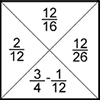### Fractions Jigsaw

##### Age 11 to 14 Challenge Level:

A jigsaw where pieces only go together if the fractions are equivalent.### Sum Equals Product

##### Age 11 to 14 Challenge Level:

The sum of the numbers 4 and 1 [1/3] is the same as the product of 4 and 1 [1/3]; that is to say 4 + 1 [1/3] = 4 � 1 [1/3]. What other numbers have the sum equal to the product and can this be so. . . .### Diminishing Returns

##### Age 11 to 14 Challenge Level:

How much of the square is coloured blue? How will the pattern continue?### Special Sums and Products

##### Age 11 to 14 Challenge Level:

Find some examples of pairs of numbers such that their sum is a factor of their product. eg. 4 + 12 = 16 and 4 × 12 = 48 and 16 is a factor of 48.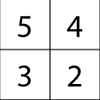### Magic Matrix

##### Age 7 to 11 Challenge Level:

Find out why these matrices are magic. Can you work out how they were made? Can you make your own Magic Matrix?### Keep it Simple

##### Age 11 to 14 Challenge Level:

Can all unit fractions be written as the sum of two unit fractions?### Ben's Game

##### Age 11 to 16 Challenge Level:

Ben, Jack and Emma passed counters to each other and ended with the same number of counters. How many did they start with?### Smaller and Smaller

##### Age 7 to 14 Challenge Level:

Can you predict, without drawing, what the perimeter of the next shape in this pattern will be if we continue drawing them in the same way?### Blue and White

##### Age 11 to 14 Challenge Level:

Identical squares of side one unit contain some circles shaded blue. In which of the four examples is the shaded area greatest?